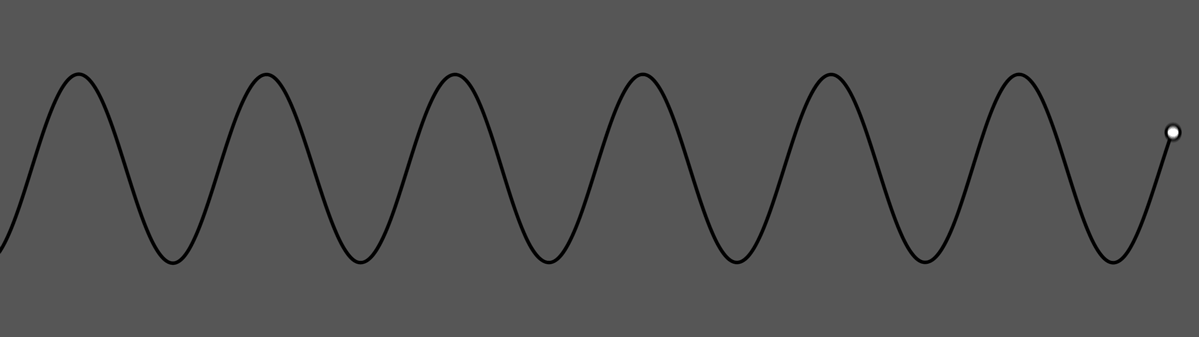Method

This node generates numeric values on the basis of mathematical pattern functions, from smooth sine waves to noise.

The node provides a preview of the pattern value that will be generated with a tracer line showing the place of the play head.Attributes

Name Description
Current Value Shows the current value of the effect. Cannot be directly changed.
Math Function The mathematical function to be used by the modifier.
• Sine, a simple sine wave.
• Cosine, a simple cosine wave.
• Interpolated Noise, random noise is generated with values and smoothly interpolated.
• Square, Jumps between 0 and 1 at a constant pace.
• Square – Inverted, Jumps between 0 and 1 at a constant pace, at opposite timing to square.
• Random Noise, Random values are generated at regular intervals with no interpolation between values.
• Perlin Noise, Noise is generated based on a gradient function, leading to smoother looking noise.
• Saw, Value Rises consistently, before sharply decreasing.
• Triangle, Value rises then decreases at the same consistent rate.
Blend Amount Control how much the modifier affects the values its input into.
Scale The scale/range of the modifier
Offset Applies an offset (post scale) to the calculated value
Speed The speed at which the value moves through the generated pattern.
Time Offset Offsets the time the value is modified from the time the waveform is played.
Time Mode How the modifier updates with relation to the time code.
• Locked To Timecode, The modifier values are locked to the time code and the same time will always yield the same numerical value.
• Running / Looping, The modifier values are disconnected from the time code and will loop seamlessly at the end of the layer. This also means that animated speed values will change smoothly.
Operation The operation to be performed on the targeted parameter.
• Add, adds to the parameter value.
• Subtract, subtracts from the parameter value.
• Multiply, multiplies the parameter value.
• Replace, replaces the parameter value.
Restore Values Toggle whether the values restore to 0. If unchecked, generated values incrementally add to the modifier value.
Use Absolute Value (Ignore Sign) Ignore negative signs.
Perlin Noise Octaves The granularity of the noise.

Inputs

There are no inputs for this node.

Outputs

The output is a numeric value that acts upon a connected parameter input of another node, using the operation method selected.